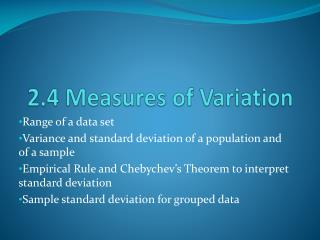DownloadDownload Presentation2.4 Measures of Variation

# 2.4 Measures of Variation

Télécharger la présentation## 2.4 Measures of Variation

- - - - - - - - - - - - - - - - - - - - - - - - - - - E N D - - - - - - - - - - - - - - - - - - - - - - - - - - -
##### Presentation Transcript

1. 2.4 Measures of Variation Range of a data set Variance and standard deviation of a population and of a sample Empirical Rule and Chebychev’s Theorem to interpret standard deviation Sample standard deviation for grouped data

2. Range The range of a data set is the difference between the maximum and minimum data entries in the set. To find the range, the data must be quantitative. Range = (Maximum data entry) – (Minimum data entry)

3. Try it yourself 1 • Finding the Range of a Data Set Find the range of the starting salaries for Corporation B. Starting Salaries for Corporation B (1000s of dollars) Range = (Maximum salary) – (Minimum salary) = 58 – 23 = 35

4. Deviation The deviation of an entry x in a population data set is the difference between the entry and the mean µ of the data set. Deviation of x = x - µ

5. Try it yourself 2 • Finding the Deviations of a Data Set Find the deviation of each starting salary for Corporation B given in Example 1. Starting Salaries for Corporation B (1000s of dollars)

6. Try it yourself 2

7. Population variance The population varianceof a population data set of N entries is Population variance = The symbol σ is the lowercase Greek letter sigma.

8. Population standard deviation The population standard deviation of a population data set of N entries is the square root of the population variance. Population standard deviation = σ =

9. Try it yourself 3 • Finding the Population Standard Deviation Find the population variance and standard deviation of the starting salaries for Corporation B given in Example 1. Starting Salaries for Corporation B (1000s of dollars)

10. Try it yourself 3 SSx= 1102.5 N = 10 σ² = 1102.5/10 ≈ 110.3 σ = √(1102.5/10) ≈ 10.5

11. Sample variance and sample standard deviation The sample varianceand sample standard deviation of a sample data set of n entries are listed below. Sample variance = s² = Sample standard deviation = s =

12. Symbols in Variance and Standard Deviation Formulas

13. Try it yourself 4 • Finding the Sample Standard Deviation Find the sample standard deviation of the starting salaries for the Chicago branch of Corporation B. Starting Salaries for Corporation B (1000s of dollars) SSx = 1102.5 n = 10 s² = 1102.5/9 ≈ 122.5 s = √(1102.5/9) ≈ 11.1

14. Try it yourself 5 • Using Technology to Find the Standard Deviation Sample office rental rates (in dollars per square foot per year) for Seattle’s central business district are listed. Use a calculator or a computer to find the mean rental rate and the sample standard deviation. 40.00 43.00 46.00 40.50 35.75 39.75 32.75 36.75 35.75 38.75 38.75 36.75 38.75 39.00 29.00 35.00 42.75 32.75 40.75 35.25 Sample mean = 37.89 Sample standard deviation = 3.98

15. Try it yourself 6 Write a data set that has 10 entries, a mean of 10, and a population standard deviation that is approximately 3. (There are many correct answers.) 7, 7, 7, 7, 7, 13, 13, 13, 13, 13

16. Empirical Rule

17. Try it yourself 7 • Using the Empirical Rule In a survey conducted by the National Center for Health Statistics, the sample mean height of women in the United States (ages 20-29) was 64.3 inches, with a sample standard deviation of 2.62 inches. Estimate the percent of women’s heights that are between 64.3 and 66.92 inches tall. The height of 66.92 inches is 1 standard deviation away from the mean which is 64.3. According to the Empirical Rule, this is approximately 34% of the data. Therefore, approximately 34% of women ages 20-29 are between 64.3 and 66.92 inches tall.

18. Chebychev’s Theorem

19. Try it yourself 8 • Using Chebychev’s Theorem The age distributions for Alaska and Florida are shown in the histograms. Decide which is which. Apply Chebychev’s Theorem to the data for Alaska using k = 2. What can you conclude? μ = 31.6 μ = 39.2 σ = 19.5 σ = 24.8

20. Try it yourself 8 Apply Chebychev’s Theorem to the data for Alaska using k = 2. What can you conclude? μ = 31.6 σ = 19.5 At least 75% of the data lie within 2 standard deviations of the mean. Therefore, at least 75% of the population of Alaska is between 0 and 70.6 years old.

21. Standard Deviation for Grouped Data In Section 2.1, you learned that large data sets are usually best represented by frequency distributions. The formula for the sample standard deviation for a frequency distribution is Sample standard deviation = s = where n = ∑f is the number of entries in the data set.

22. Try it yourself 9 • Finding the Standard Deviation for Grouped Data Find the sample mean and sample standard deviation of the data set.

23. Try it yourself 9 Sample mean = 1.7 Sample standard deviation = 1.5

24. Try it yourself 10 • Using Midpoints of Classes The circle graph shows the results of a survey in which 1000 adults were asked how much they spend in preparation for personal travel each year. Make a frequency distribution for the data. Then use the table to estimate the sample mean and the sample standard deviation of the data set.

25. Try it yourself 10 Sample mean = 188.5 Sample standard deviation ≈ 151.91 So, the sample mean is \$188.50 per year, and the sample standard deviation is about \$151.91 per year.